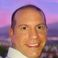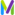cancel
Showing results for
Show  only  | Search instead for
Did you mean:

## Calculating new metric from existing metricsParticipant

In Dynatrace we have Temperature metrics per each device. There are multiple devices in one room and the room is a metric dimension.
I'm able to create a query in Data Explorer to show the average temperature per room.

How can I create a new metric called room_average based on a query from Data Explorer in Dynatrace?Hi @mariusz_cwiklin As you indicate, it is possible to do operations between metrics and obtain the ones you are looking for in the Data Explorer editor, but AFAIK it is not possible to create a metric from metrics.

Metric expressions enable you to apply simple arithmetic operations on operands (metrics or numbers).

For example, this expression calculates the ratio (as a percentage) of two metrics:
((metric1)/(metric2))*(100)

Building on the example above, we have the following basic components to work with:

• Operand: a metric or number
• Parentheses: ()
• Arithmetic operators: +, -, *, /
• Negation: -()
The true delight is in the finding out rather than in the knowing.